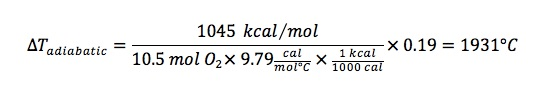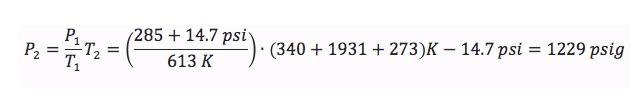## ESP Energetics Calculation

This calculation is based on the Dow Lab Safety Module entitled, “Energy Calculations.”

The consequences of not considering the potential energy release of a chemical system includes:

• injury
• loss of primary containment
• equipment failure
• laboratory damage

Consider the example of pressure in a reaction vessel.  A researcher is going to study the selective oxidation of 1 mole of o-xylene in a CSTR at 340°C and 285 pig.  The reactor has a pressure rating of 2950 pig and a rupture disk with a burst pressure of 2000 psig.  Is this relief device necessary?

There are three reactions that occur in this system.

• oX (o-xylene) + 3 O2 → PA (phthalic anhydride),  ΔHrxn = -264 kcal/mol
• PA + 7.5 O2 → 8 CO2 + 2 H2O,  ΔHrxn = -781 kcal/mol
• oX + 10.5 O2 → 8 CO2 + 5 H2O,  ΔHrxn = -1045 kcal/mol

The last reaction represents the worst case scenario (i.e., the largest heat release if oX undergoes total combustion), and is thus used to calculate a reasonable margin of safety.

Using the worst case scenario, estimate the adiabatic temperature rise:

where

• nA ≡ total moles of reactant in the system
• Cp ≡ heat capacity of the reaction mixture
• XA ≡ conversion (which we assume is 100%)
• Qadiabatic ≡ heat of reaction

Substituting values:Using a ideal gas equation of state, an estimate of pressure rise can then be made:This result suggests a 2000 psig rupture disk setting in a device with a 2950 psig pressure rating represents a relatively safe margin of error.

Watch the video on the Dow safety website.